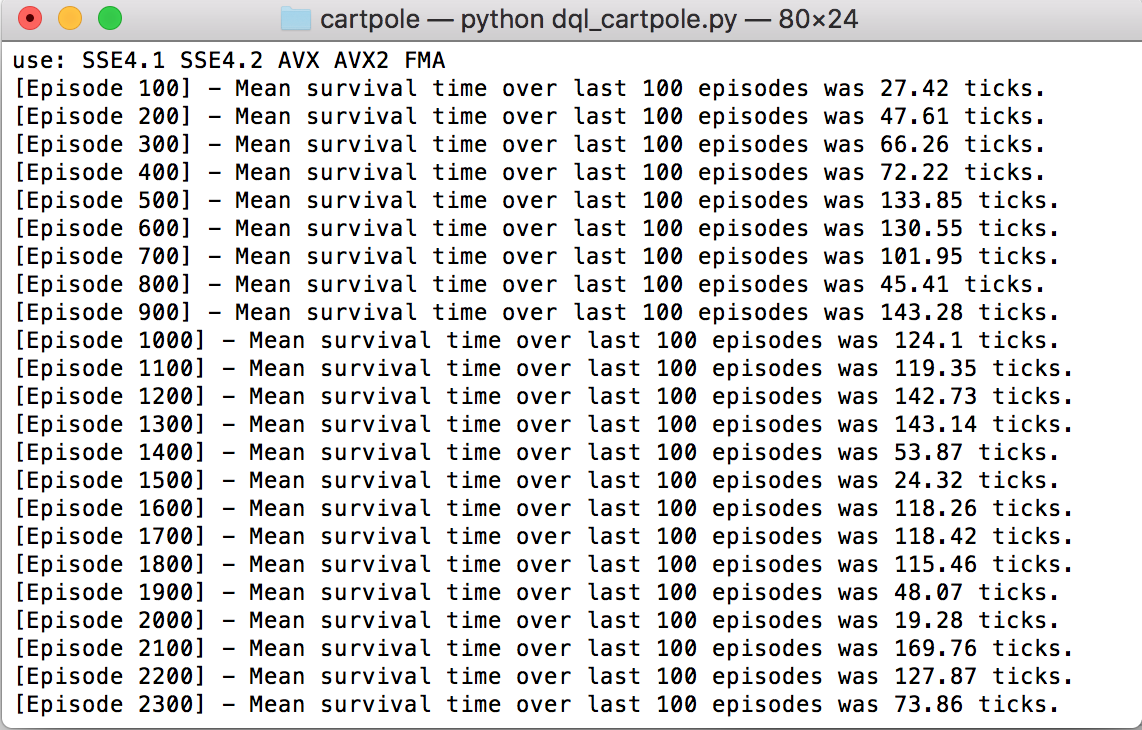Instantly share code, notes, and snippets.

#n1try/dqn_cartpole.py Last active Dec 27, 2019

 # Inspired by https://keon.io/deep-q-learning/ import random import gym import math import numpy as np from collections import deque from keras.models import Sequential from keras.layers import Dense from keras.optimizers import Adam class DQNCartPoleSolver(): def __init__(self, n_episodes=1000, n_win_ticks=195, max_env_steps=None, gamma=1.0, epsilon=1.0, epsilon_min=0.01, epsilon_log_decay=0.995, alpha=0.01, alpha_decay=0.01, batch_size=64, monitor=False, quiet=False): self.memory = deque(maxlen=100000) self.env = gym.make('CartPole-v0') if monitor: self.env = gym.wrappers.Monitor(self.env, '../data/cartpole-1', force=True) self.gamma = gamma self.epsilon = epsilon self.epsilon_min = epsilon_min self.epsilon_decay = epsilon_log_decay self.alpha = alpha self.alpha_decay = alpha_decay self.n_episodes = n_episodes self.n_win_ticks = n_win_ticks self.batch_size = batch_size self.quiet = quiet if max_env_steps is not None: self.env._max_episode_steps = max_env_steps # Init model self.model = Sequential() self.model.add(Dense(24, input_dim=4, activation='tanh')) self.model.add(Dense(48, activation='tanh')) self.model.add(Dense(2, activation='linear')) self.model.compile(loss='mse', optimizer=Adam(lr=self.alpha, decay=self.alpha_decay)) def remember(self, state, action, reward, next_state, done): self.memory.append((state, action, reward, next_state, done)) def choose_action(self, state, epsilon): return self.env.action_space.sample() if (np.random.random() <= epsilon) else np.argmax(self.model.predict(state)) def get_epsilon(self, t): return max(self.epsilon_min, min(self.epsilon, 1.0 - math.log10((t + 1) * self.epsilon_decay))) def preprocess_state(self, state): return np.reshape(state, [1, 4]) def replay(self, batch_size): x_batch, y_batch = [], [] minibatch = random.sample( self.memory, min(len(self.memory), batch_size)) for state, action, reward, next_state, done in minibatch: y_target = self.model.predict(state) y_target[action] = reward if done else reward + self.gamma * np.max(self.model.predict(next_state)) x_batch.append(state) y_batch.append(y_target) self.model.fit(np.array(x_batch), np.array(y_batch), batch_size=len(x_batch), verbose=0) if self.epsilon > self.epsilon_min: self.epsilon *= self.epsilon_decay def run(self): scores = deque(maxlen=100) for e in range(self.n_episodes): state = self.preprocess_state(self.env.reset()) done = False i = 0 while not done: action = self.choose_action(state, self.get_epsilon(e)) next_state, reward, done, _ = self.env.step(action) next_state = self.preprocess_state(next_state) self.remember(state, action, reward, next_state, done) state = next_state i += 1 scores.append(i) mean_score = np.mean(scores) if mean_score >= self.n_win_ticks and e >= 100: if not self.quiet: print('Ran {} episodes. Solved after {} trials ✔'.format(e, e - 100)) return e - 100 if e % 100 == 0 and not self.quiet: print('[Episode {}] - Mean survival time over last 100 episodes was {} ticks.'.format(e, mean_score)) self.replay(self.batch_size) if not self.quiet: print('Did not solve after {} episodes 😞'.format(e)) return e if __name__ == '__main__': agent = DQNCartPoleSolver() agent.run()

### RossMelbourne commented Dec 18, 2017

 When I run this script I am getting 'Did not solve after 999 episodes'. I have tried using TensorFlow 1.3 and 1.4.1 using Python 3.6.3. Any suggestions why I am not getting the results you are seeing?

### JenZhao commented Jan 12, 2018

 When I ran it locally it took around 1000 episodes to solve. tensorflow-gpu==1.4.1, python 3.6.0.

### gstenger98 commented Mar 31, 2018

 I ran this locally as well, and it has never succeeded in under 1000 episodes. The code is solid, and the method looks great. Overall, it's a great project. I just don't think that this should be #1 on the leaderboard of best CartPole-v0 performances.### GarrisonD commented Apr 9, 2018

 @gstenger98 but nevertheless it was able to solve it in 85 episodes according to https://github.com/openai/gym/wiki/Leaderboard#cartpole-v0. And I believe that the matter is in versions of libraries you use while trying to reproduce the results.

### GarrisonD commented Apr 9, 2018

 @gstenger98 also I noticed that it's a bit unstable, sometimes NN can find the right weights in < 500 episodes, and sometimes it can learn just nothing in >= 1000 episodes - I had the case when there wasn't any reward greater than 25 through all 1000 episodes.

### sungsulim commented Apr 23, 2018

 Perhaps it's unstable because it is not using target networks?

### rakeshmallick commented Apr 30, 2018 • edited

 I ran this programme got did not solve after 999 episodes.